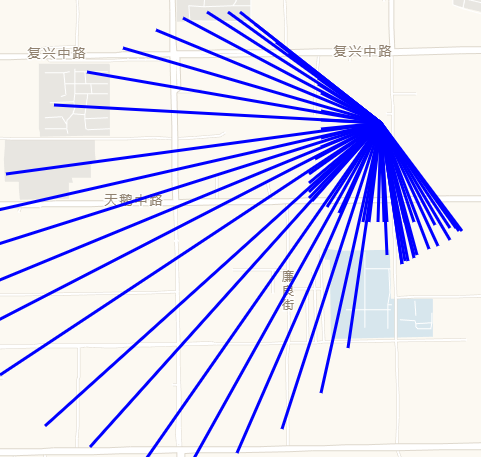# 在高德地图上用svg.js绘制简单图形

2019/04/10 10:10

## 一、高德地图

``````<script type="text/javascript" src="https://webapi.amap.com/maps?v=1.4.15&key=申请的key"></script>
``````

``````<div id="container"></div>
``````
``````html, body, #container {
margin: 0;
width: 100%;
height: 100%;
}
``````

``````// 第一个参数是容器名称，第二个参数可以按自己需求随意配置。
var map = new AMap.Map('container', {
zoom: 15, // 缩放等级
center: [115.49481017, 38.88656455], // 中心点
});
``````

## 二、自定义图层

``````map.on('complete', function(){
// TODO 编写自定义图层
})
``````

`svg`和地图类似，也需要先声明一个容器，此容器和地图等宽等高

``````var svg = \$('<svg id="drawing" xmlns="http://www.w3.org/2000/svg" xmlns:xlink="http://www.w3.org/1999/xlink" version="1.1" ></svg>');
``````
``````#drawing {
margin: 0;
width: 100%;
height: 100%;
}
``````

``````// 第一个参数传入我们创建的svg对象，第二个参数为图层配置，可以根据自己需求进行配置
var customLayer = new AMap.CustomLayer(svg, {
zIndex: 100,
zooms: [3, 18],
alwaysRender: true
});
``````

## 二、在图层中画自己喜欢的图形。

``````// 调用svg.js提供的SVG函数，传入我们创建的svg对象，创建所需要的draw对象。
var draw = SVG(svg);
``````

``````var isDraw = false; // 用来判断是开始画线还是结束画线。
var startPix; // 用来存储起点
map.on('click', function(ev){
isDraw = !isDraw;
if(isDraw) {
// isDraw为true就标明起点
startPos = ev.pixel;
}else{
// isDraw为false就画线。
}
})
``````

``````function drawLine(start, end) {
var lineWth = 3;
var lineColor = 'blue';
var x = start.x;
var y = start.y;
var x1 = end.x;
var y1 = end.y;
line = draw.line(x, y, x1, y1).stroke({ color: lineColor, width: lineWth });
return line;
}
``````

``````map.on('click', function(ev){
isDraw = !isDraw;
if(isDraw){
// isDraw为true就标明起点
startPix = ev.pixel;
}else{
// isDraw为false就画线。
var endPix = ev.pixel;
drawLine(startPix, endPix);
}
})
``````

``````map.on('mousemove', function (ev) {
if(isDraw) {
drawLine(startPix, ev.pixel)
}
});
````````````var lineGroup; // 声明一个对象 存储line。
map.on('click', function(ev){
isDraw = !isDraw;
if(isDraw){
// isDraw为true就标明起点
startPix = ev.pixel;
lineGroup = draw.group(); // 开始画线时创建一个group。
}else{
// isDraw为false就画线。
var endPix = ev.pixel;
drawLine(startPix, endPix);
}
});
map.on('mousemove', function(ev){
if(isDraw) {
lineGroup.clear(); // 在鼠标移动时先将group清空。
var line = drawLine(startPix, ev.pixel);
}
})
``````

## 三、地图重绘时，让画的线随实际经纬度坐标重绘。

``````var positions = [];
var startPos;
``````

``````map.on('click', function(ev){
isDraw = !isDraw;
if(isDraw){
// isDraw为true就标明起点
startPos = ev.lnglat; /*手动高亮*/
startPix = ev.pixel;
lineGroup = draw.group();
}else{
// isDraw为false就画线。
var endPix = ev.pixel;
drawLine(startPix, endPix);
positions.push({ /*手动高亮*/
start: startPos,
end: ev.lnglat
})
}
});
``````

``````customLayer.render = onRender;
function onRender() {
draw.clear(); // 先将画板清空
for(var i = 0;i < positions.length;i++){
// 需要将经纬度坐标转换为容器内坐标，lngLatToContainer是高德提供的转换方法
var startPixCon = map.lngLatToContainer(positions[i].start);
var endPixCon = map.lngLatToContainer(positions[i].end);
drawLine(startPixCon, endPixCon);
}
}
``````

0
0 收藏

### 作者的其它热门文章0 评论
0 收藏
0